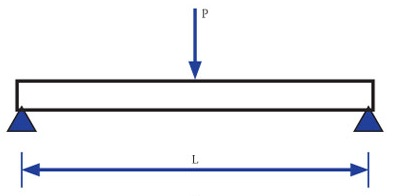A Beam may be loaded in a variety of ways. For the analysis purpose it may be splitted in three categories.

A concentrated load is the one which acts over a small length that it is assumed to act at a point. Practically, a point load cannot be places as knife edge contact but for calculation purpose we consider that load is being transmitted at a point.A distributed load acts over a finite length of the beam. A distributed load  may be uniformly. Such loads are measured by their intensity which is expressed by the force per unit distance along the axis of the beam.A load is spread over a beam in such a way that it varies uniformly on each unit length, is known as uniformly varying load. Sometimes, the load is zero at one end and increases uniformly to the other. such load is known as triangular load.In other Words,

A uniformly varying load implies that the intensity of loading increases or decreases at a constant rate along the length.Related Post: